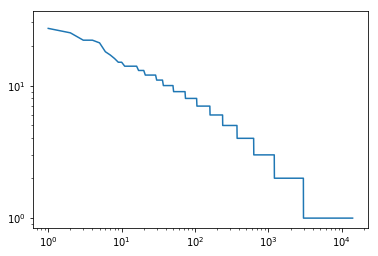• 优先连接性（Preferential attachment）/马太效应
• “凡有的，还要加给他，叫他有余；没有的，连他所有的也要夺过来。”
• 成长性
• 网络的尺度不受客观条件的限制，可以无限增长

Youtube上有个视频对幂律分布讲得很清楚，对其中提到的两个实验，我利用python进行了模拟。模拟中使用的jupyter notebook文件放在了github gist上。

## 猴子和打字机

import string,random
from collections import Counter
s=string.ascii_lowercase+" "
s="abcd "
monkey=''.join(random.choices(s, k=10000000))
monkey=[m for m in monkey.split(" ") if m]
c=Counter(monkey)
common=c.most_common()[:10000]
fre=[value for (key,value) in common]
plt.plot(fre)
plt.xscale('log')
plt.yscale('log')
plt.show()


## 连接曲别针，第一种方法

l=100
list=np.ones(l)
for i in range(100000):
s=sum(list)
p=[j/s for j in list]
k=np.random.choice(l,1, p=p)
list[k]+=1
plt.plot(sorted(list,reverse=True))
plt.xscale('log')
plt.yscale('log')
plt.show() #指数分布


## 连接曲别针，the right way

tic=timeit.default_timer()
l=20000
list=*l
while len(list)>14000:
s=sum(list)
p=[j/s for j in list]
k1=np.random.choice(len(list),1, p=p)
a1=list.pop(k1)
s=sum(list)
p=[j/s for j in list]
k2=np.random.choice(len(list),1, p=p)
a2=list.pop(k2)
list.append(a1+a2)
toc=timeit.default_timer()
print(toc-tic)
plt.plot(sorted(list,reverse=True))
plt.xscale('log')
plt.yscale('log')
plt.show()2018年4年3日的编辑：

1. 这似乎和Net neutrality并不是一回事。并没有什么邪恶组织，邪恶的只有系统而已。 ↩︎